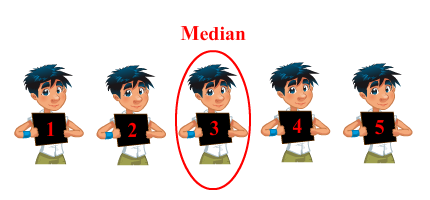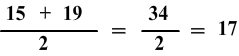# Median

Print Rate 0 stars
Lesson size:
Message preview:
Someone you know has shared lesson with you:

To play this lesson, click on the link below:

https://www.turtlediary.com/lesson/median.html

Hope you have a good experience with this site and recommend to your friends too.

Login to rate activities and track progress.
Login to rate activities and track progress.

The median is the number that lies exactly the halfway of the given set, when the data is arranged in either ascending or descending order.

### Example

• Take the set: 1, 2, 3, 4, 5.

Here the median is 3. This set is already written in order from least to greatest, so all we have to do is find the middle number.

1, 2, 3, 4, 5## Median for unordered set of odd numbers

Take the set: 38, 14, 44, 26, 28

The first thing we need to do is put the numbers in order from lowest to highest starting with 14 and ending with 44:

14, 26, 28, 38, 44

The middle number is 28.

So, median of the given data is 28.

## Median for unordered set of even numbers

Take the set: 15, 19, 8, 31

The first thing we will do is to arrange the data from least to greatest.

The number of elements in given set is even i.e. four. We will consider only the two numbers that are in the middle:

8, 15, 19, 31

In such case, we take the two middle numbers 15 and 19 and find their mean.

So, median of the given data is 17.## Median

• The median is the number that lies exactly the half way of the given set, when the data is arranged in either ascending or descending order.
• To find the median of unordered set of odd numbers, we first arrange the data in ascending or descending order and find the middlemost number.
• To find the median of unordered set of even numbers, we first arrange the data in ascending or descending order and find the two middle numbers. Median is the average or mean of those two numbers.

## Similar Lessons

Become premium member to get unlimited access.